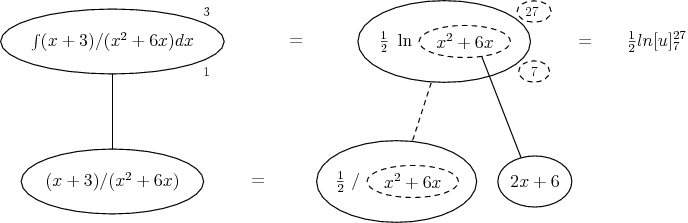# Thread: Integration of Logaritmic Functions

1. ## Integration of Logaritmic Functions

$\displaystyle \int_1^3 (x+3)/(x^2+6x) dx = ?$

I tired separating and simplifying the problem into two different integrals:

$\displaystyle \int_1^3 1/(x+6)$ +$\displaystyle \int_1^3 3/(x^2+6x)$

and get...
$\displaystyle \int_1^3 ln(x+6) + \int_1^3 3ln(x^2+6x)$

evaluating I get

$\displaystyle [ln9-ln7]+ 3[ln27+ln7]$

but that is not any of the solutions.2. Try Integration by Substitution, which is basically the Chain Rule backward:

$\displaystyle \int\,f'(g(x))g'(x)\,dx\,=\,f(g(x))\,+\,C$

Letting $\displaystyle u\,=\,g(x)\,=\,x^2\,+\,6x$, we can see that $\displaystyle du\,=\,g'(x)\,dx\,=\,(2x\,+\,6)\,dx\,=\,2(x\,+\,3) \,dx$, so that the integral becomes

$\displaystyle \int_{u\,=\,7}^{u\,=\,27}\,\frac{1}{2u}\,du.$

Hope this helps.

3. Natural log is supposed to be in the answer...any ideas?

4. Hi frog09,

Do you understand what Scott H says in #2? He has almost completely worked the problem for you.

Cant you figure out what $\displaystyle \int_{u\,=\,7}^{u\,=\,27}\,\frac{1}{2u}\,du$ is ?

If you integrate the above you will see the "supposed natural logs"5. ##yes! a moment of enlightenment. thanks!6.Originally Posted by frog09$\displaystyle \int_1^3 (x+3)/(x^2+6x) dx = ?$

I tired separating and simplifying the problem into two different integrals:

$\displaystyle \int_1^3 1/(x+6)$ +$\displaystyle \int_1^3 3/(x^2+6x)$

and get...
$\displaystyle \int_1^3 ln(x+6) + \int_1^3 3ln(x^2+6x)$

evaluating I get

$\displaystyle [ln9-ln7]+ 3[ln27+ln7]$

but that is not any of the solutions.$\displaystyle \begin{gathered}\int\limits_1^3 {\frac{{x + 3}}{{x^2 + 6x}}dx} = \frac{1}{2}\int\limits_1^3 {\frac{{2x + 6}}{{x^2 + 6x}}dx = } \frac{1}{2}\int\limits_1^3 {\frac{{d\left( {x^2 + 6x} \right)}}{{x^2 + 6x}} = } \hfill \\= \left. {\frac{1}{2}\ln \left( {x^2 + 6x} \right)} \right|_1^3 = \frac{1}{2}\left( {\ln 27 - \ln 7} \right) = \frac{1}{2}\left( {3\ln 3 - \ln 7} \right). \hfill \\\hfill \\ \end{gathered}$

7. Are you sure? I thought we had to change the bounds of integration after the change of variables.

Frog09, remember also that $\displaystyle \ln\,u$ is defined as $\displaystyle \int_1^u\,\frac{1}{x}\,dx.$

8. Except that he didn't actually change the variable, so it was ok. This is how I would keep track of it...(As usual straight continuous lines diff or anti-diff with respect to x, straight dashed lines with respect to the dashed balloon expression.)

Don't integrate - balloontegrate! Balloon Calculus: worked examples from past papers

9.Originally Posted by Scott HAre you sure? I thought we had to change the bounds of integration after the change of variables.

Frog09, remember also that $\displaystyle \ln\,u$ is defined as $\displaystyle \int_1^u\,\frac{1}{x}\,dx.$
Yes, I am sure!
In my solution we must not change the bounds of integration.
Because

$\displaystyle \left. {\int\limits_b^a {\frac{{f'\left( x \right)}}{{f\left( x \right)}}dx = } \ln \left( {f\left( x \right)} \right)} \right|_b^a = \ln \left| {f\left( a \right)} \right| - \ln \left| {f\left( b \right)} \right|.$

10. All right, I see.

#### Search Tags

functions, integration, logaritmic